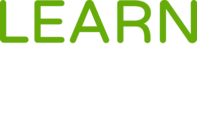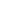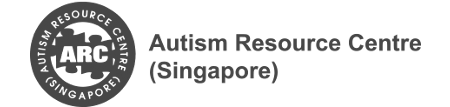#Current of Electricity is a Course

## Current of Electricity

Started Nov 8, 2017

### Full course description

This course provides knowledge to support learners with Autism Spectrum Disorder (ASD) in Current Electricity.

The section begins with a discussion of electric charges that are moving. Next, we build on our understanding on moving electric charges and relate them to the concepts of current, voltage and resistance. We also study how these concepts are applied to simple circuits and household electricity.

This course contains four modules:

• Electric Current
• Electromotive Force and Potential Difference
• Resistance
• Resistivity

By the end of this course, you will be able to:

• State that current is the rate of flow of charge and is measured in amperes (A)
• Distinguish between conventional current and electron flow
• Recall and apply the relationship charge = current x time to new situations or to solve related problems
• Draw electric circuit diagrams
• Define electromotive force (e.m.f.) as the work done by a source in driving unit charge around a complete circuit
• Calculate the e.m.f. where several sources are arranged in series
• State that the e.m.f. of a source and the potential difference (p.d.) across a circuit component are measured in volts
• Define the p.d. across a component in a circuit as the work done in moving unit charge through the component
• State the definition that resistance = p.d./ current
• Apply the relationship R=V/I to new situations or to solve related problems
• Describe an experiment to determine resistance of a metallic conductor using a voltmeter and an ammeter, and making the necessary calculations
• State Ohm’s Law
• Describe the effect of temperature increase on the resistance of a metallic conductor
• Sketch and interpret the I-V characteristic graphs for a metallic conductor at constant temperature, for a filament lamp and for a semi-conductor diode
• State that the resistance R of a wire varies proportionally with the length l and is inversely proportional to the cross sectional area A of the wire

This course is available to all Pathlight School students.

Pathlight School students can enrol in this course. Login to Learn for Life eCampus is required.## Physics

Pathlight School

### Offered By5-outcomes-cognition, for-students, for-educators, current, electricity, general-knowledge, academic-bridging, science, voltage, power